# How to make your own blocks with code! (Introduction to S-Functions)

I have to admit... before joining MathWorks, I was afraid of S-Functions.

Now this makes me laugh, because I know that writing an S-Function is very powerful and not that complicated.

In my opinion, understanding S-Functions is the best way to understand how Simulink works.

When looking at the previous posts on this blog, I realized that I wrote a few posts about advanced usage of s-functions (Variable Size Signals and Unit Delay and Scheduling Future Events), but I never covered the basic of how S-Functions work.

Getting Started with S-Functions

For most MATLAB users, I recommend starting by writing S-Functions in MATLAB (in opposite to C or C++). MATLAB S-Functions have some limitations compared to C or C++, but debugging is easier.

sfundemos

This will launch a library containing links to many examples. In this collection, the simplest model to start with is msfcndemo_timestwo.mdl.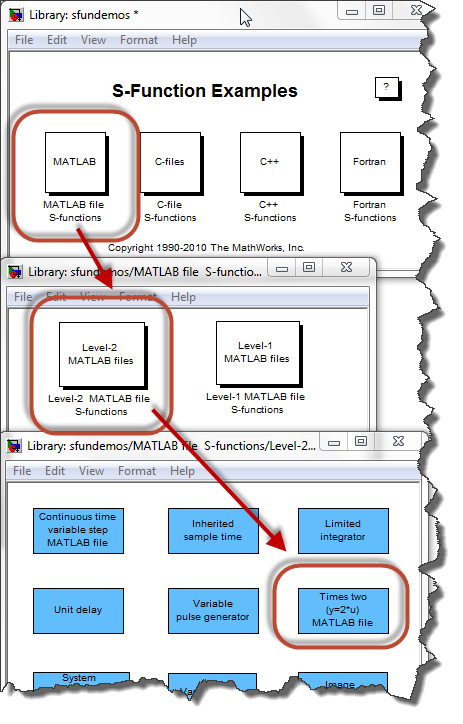In this model, click on the annotation to open the source code of the S-Function.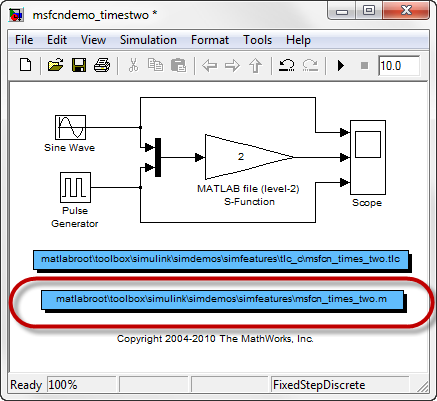Basic structure of an S-Function

If you look at the code, you will find that the S-Function is in fact just a MATLAB function, taking as input a variable block and passing it to another function using the line setup(block).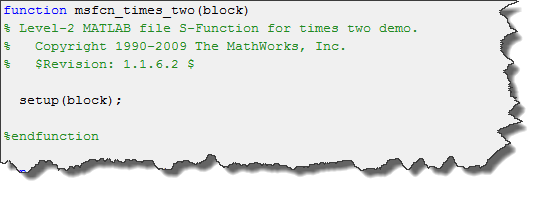The block variable

This block variable is an instance of the Simulink.MSFcnRunTimeBlock class. We call it the S-Function run-time object.

Using the run-time object, the S-Function can exchange information with the Simulink engine.

The setup(block) function

In the setup function, the run-time object allows you to specify and obtain information about various characteristics of the block, including ports, parameters, states, work vectors, etc.

In our example msfcn_times_two.m, the properties of ports are set using lines like: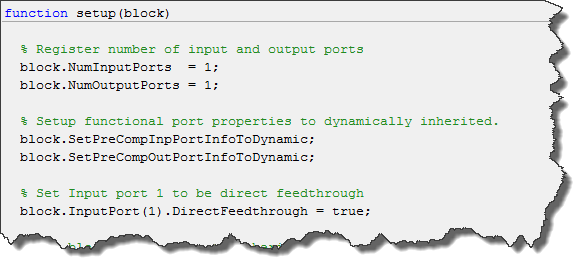Also, the setup function is responsible for registering a set of callback methods that the Simulink solver will call during different phases of the simulation.

In the times two example, we register only one method, the output function: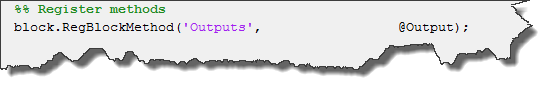If you are interested to see the list of available methods and when they are called, I recommend going through the documentation section How the Simulink Engine Interacts with C S-Functions

Registered Methods

Every time the Simulink engine calls a method of an S-Function, it passes to it the run-time object.

In msfcn_times_two.m, we access the data of the input port and use it to compute the value that will be written to the output port: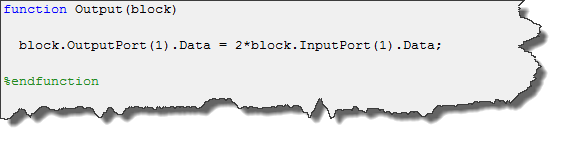Conclusion

This is it for this simple s-function example.

Of course, if you need to multiply a signal by two in Simulink, I recommend using a Gain block, and not an S-Function. But hopefully this simple example gives you an idea of how MATLAB S-functions work.